# Bash Scripting – For Loop

Since BASH is a command-line language, we get some pretty feature-rich experience to leverage the programming skills to perform tasks in the terminal. We can use loops and conditional statements in BASH scripts to perform some repetitive and tricky problems in a simple programmatic way. In this article, we are going to focus on the for loop in BASH scripts.

Depending on the use case and the problem it is trying to automate, there are a couple of ways to use loops.

• Simple For loop
• Range-based for loop
• Array iteration for loops
• C-Styled for loops
• Infinite for loop

## Simple For loop

To execute a for loop we can write the following syntax:

`#!/bin/bashfor n in a b c;do   echo \$ndone`
• In the first iteration, `n` takes the value “a”, and the script prints “a”.
• In the second iteration, `n` takes the value “b”, and the script prints “b”.
• In the third iteration, `n` takes the value “c”, and the script prints “c”.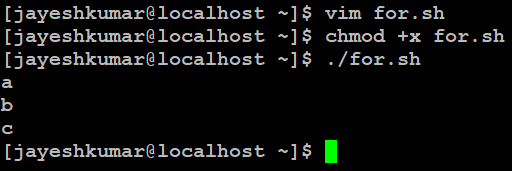The above command will iterate over the specified elements after the in keyword one by one. The elements can be numbers, strings, or other forms of data.

## Range-based for loop

We can use range-based for loops. In this type of loop, we can specify the number to start, to stop, and to increment at every iteration(optional) in the statement. There are two ways you can do this i.e. by mentioning the increment/decrementer value and by incrementing by one by default. The syntax looks like this:

`#!/bin/bashfor n in {1..5}; do    echo \$ndone`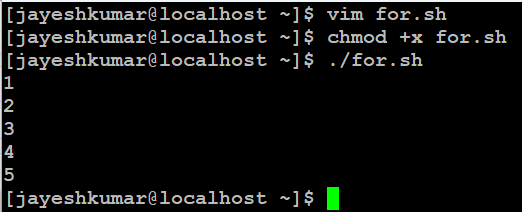In the above code, we use the “{}” to specify a range of numbers. Inside the curly braces, we specify the start point followed by two dots and an endpoint. By default, it increments by one. Hence we print 5 numbers from 1 to 5 both inclusive.

`#!/bin/bashfor n in {1..5..2}; do    echo \$ndone`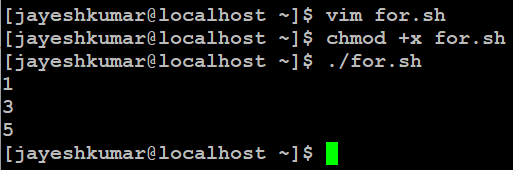Here we can see that the loop incremented by 2 units as mentioned in the curly braces. Thus, this makes working with numbers very easy and convenient. This can also be used with alphabetical characters.

NOTE: We cannot use variables inside the curly braces, so we will have to hardcode the values. To use the variables, we see the traditional C-styled for loops in the next few sections.

## Array iteration for loops

We can iterate over arrays conveniently in bash using for loops with a specific syntax. We can use the special variables in BASH i.e @ to access all the elements in the array. Let’s look at the code:

`#!/bin/bashs=("football" "cricket" "hockey") for n in \${s[@]}; do    echo \$ndone`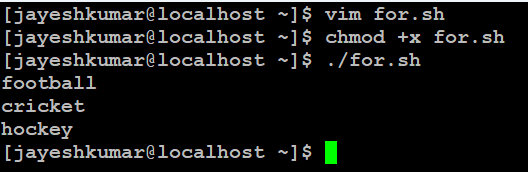We can iterate over the array elements using the @ operator that gets all the elements in the array. Thus using the for loop we iterate over them one by one. We use the variable \${variable_name[@]} in which, the curly braces here expand the value of the variable “s” here which is an array of strings. Using the [@] operator we access all the elements and thus iterate over them in the for a loop. Here, the “n” is the iterator hence, we can print the value or do the required processing on it.

## C-Styled for loops

As said earlier, we need to use the variables inside the for loops to iterate over a range of elements. And thus, the C-styled for loops play a very important role. Let’s see how we use them.

`#!/bin/bashn=7for (( i=1 ; i<=\$n ; i++ )); do    echo \$idone`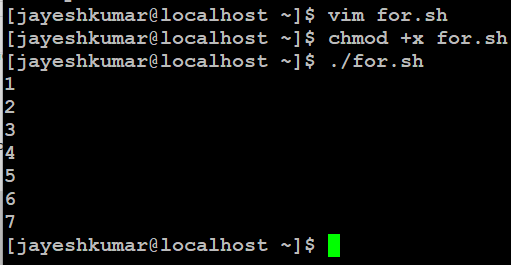As we can see we can dynamically use the value of the range of end conditions. Remember the spaces between the double braces might be intentional and are part of the syntax. C-styled for loops are the loops that have 3 parts, the initializing iterator, the incrementor/decrementer, and the end condition.

In the syntax above, we have initialized the loop iterator/counter to 1 that can be anything as per choice. The second part is the end condition, here we have used the variable “n” which is initialized before the for loop and thus we use the simple \$ operator to get the value of the variable. Finally, we have the incrementor/decrementer which changes the iterator/counter to a value that can be anything but, in the example, we have used the unary operator (++) to increment the value by one which is equivalent to “i=i+1”. Thus we can use statements like i+=2, i–,++i, and so on and so forth.

## Infinite for loop

We don’t use this often but it is sometimes useful to get certain things working. The syntax is quite easy and similar to the C-styled for loops.

`#!/bin/bashn=4for (( ; ; )); do    if [ \$n -eq 9 ];then        break    fi    echo \$n    ((n=n+1))done`

The loop starts with `n` set to 4. It increments `n` by 1 at each iteration and prints the value of `n` until `n` becomes equal to 9. When `n` reaches 9, the `break` statement is executed, and the loop terminates. The script stops after printing the numbers 4 to 8.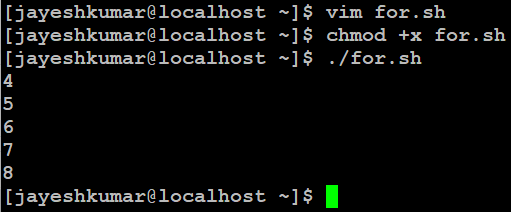As we can see the `for` loop has no conditions and this loops forever but we have a condition statement to check that it doesn’t go on forever.  We use the break statement inside the if statement so as to get out of the loop and stop iterating with the iterator. We have used the incrementor to increment the variable in the loop otherwise the loop is infinite. Of course, we need some logic to break out of the loop and that is why we need to use the if conditional statement.

Whether you're preparing for your first job interview or aiming to upskill in this ever-evolving tech landscape, GeeksforGeeks Courses are your key to success. We provide top-quality content at affordable prices, all geared towards accelerating your growth in a time-bound manner. Join the millions we've already empowered, and we're here to do the same for you. Don't miss out - check it out now!

Previous
Next